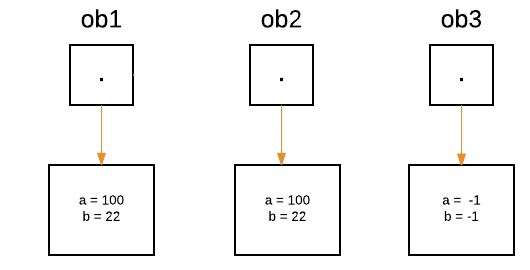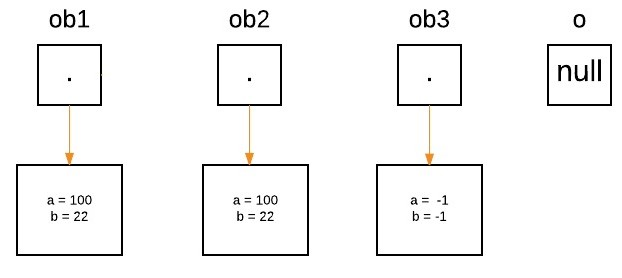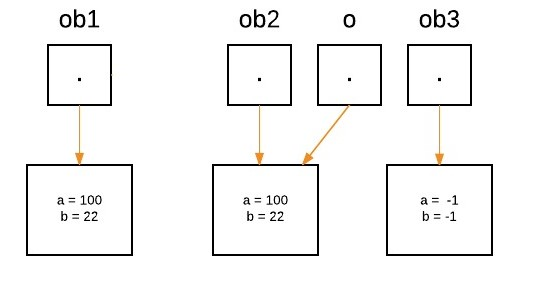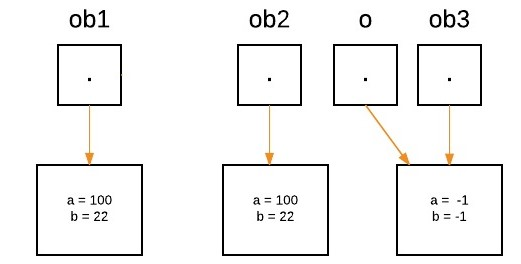Open In App

# Passing and Returning Objects in Java

Although Java is strictly passed by value, the precise effect differs between whether a primitive type or a reference type is passed. When we pass a primitive type to a method, it is passed by value. But when we pass an object to a method, the situation changes dramatically, because objects are passed by what is effectively call-by-reference. Java does this interesting thing that’s sort of a hybrid between pass-by-value and pass-by-reference.
Basically, a parameter cannot be changed by the function, but the function can ask the parameter to change itself via calling some method within it.

• While creating a variable of a class type, we only create a reference to an object. Thus, when we pass this reference to a method, the parameter that receives it will refer to the same object as that referred to by the argument.
• This effectively means that objects act as if they are passed to methods by use of call-by-reference.
• Changes to the object inside the method do reflect the object used as an argument.

Illustration: Let us suppose three objects ‘ob1’ , ‘ob2’ and ‘ob3’ are created:

```ObjectPassDemo ob1 = new ObjectPassDemo(100, 22);
ObjectPassDemo ob2 = new ObjectPassDemo(100, 22);
ObjectPassDemo ob3 = new ObjectPassDemo(-1, -1);```From the method side, a reference of type Foo with a name a is declared and it’s initially assigned to null.

`boolean equalTo(ObjectPassDemo o);`As we call the method equalTo, the reference ‘o’ will be assigned to the object which is passed as an argument, i.e. ‘o’ will refer to ‘ob2’ as the following statement execute.

`System.out.println("ob1 == ob2: " + ob1.equalTo(ob2));`Now as we can see, equalTo method is called on ‘ob1’ , and ‘o’ is referring to ‘ob2’. Since values of ‘a’ and ‘b’ are same for both the references, so if(condition) is true, so boolean true will be return.

`if(o.a == a && o.b == b)`

Again ‘o’ will reassign to ‘ob3’ as the following statement execute.

`System.out.println("ob1 == ob3: " + ob1.equalTo(ob3));`• Now as we can see, the equalTo method is called on ‘ob1’ , and ‘o’ is referring to ‘ob3’. Since values of ‘a’ and ‘b’ are not the same for both the references, so if(condition) is false, so else block will execute, and false will be returned.

In Java we can pass objects to methods as one can perceive from the below program as follows:

Example:

## Java

 `// Java Program to Demonstrate Objects Passing to Methods.` `// Class``// Helper class``class` `ObjectPassDemo {``    ``int` `a, b;` `    ``// Constructor``    ``ObjectPassDemo(``int` `i, ``int` `j)``    ``{``        ``a = i;``        ``b = j;``    ``}` `    ``// Method``    ``boolean` `equalTo(ObjectPassDemo o)``    ``{``        ``// Returns true if o is equal to the invoking``        ``// object notice an object is passed as an``        ``// argument to method``        ``return` `(o.a == a && o.b == b);``    ``}``}` `// Main class``public` `class` `GFG {``    ``// MAin driver method``    ``public` `static` `void` `main(String args[])``    ``{``        ``// Creating object of above class inside main()``        ``ObjectPassDemo ob1 = ``new` `ObjectPassDemo(``100``, ``22``);``        ``ObjectPassDemo ob2 = ``new` `ObjectPassDemo(``100``, ``22``);``        ``ObjectPassDemo ob3 = ``new` `ObjectPassDemo(-``1``, -``1``);` `        ``// Checking whether object are equal as custom``        ``// values``        ``// above passed and printing corresponding boolean``        ``// value``        ``System.out.println(``"ob1 == ob2: "``                           ``+ ob1.equalTo(ob2));``        ``System.out.println(``"ob1 == ob3: "``                           ``+ ob1.equalTo(ob3));``    ``}``}`

Output

```ob1 == ob2: true
ob1 == ob3: false```

### Defining a constructor that takes an object of its class as a parameter

One of the most common uses of object parameters involves constructors. Frequently, in practice, there is a need to construct a new object so that it is initially the same as some existing object. To do this, either we can use Object.clone() method or define a constructor that takes an object of its class as a parameter.

Example

## Java

 `// Java program to Demonstrate One Object to``// Initialize Another` `// Class 1``class` `Box {``    ``double` `width, height, depth;` `    ``// Notice this constructor. It takes an``    ``// object of type Box. This constructor use``    ``// one object to initialize another``    ``Box(Box ob)``    ``{``        ``width = ob.width;``        ``height = ob.height;``        ``depth = ob.depth;``    ``}` `    ``// constructor used when all dimensions``    ``// specified``    ``Box(``double` `w, ``double` `h, ``double` `d)``    ``{``        ``width = w;``        ``height = h;``        ``depth = d;``    ``}` `    ``// compute and return volume``    ``double` `volume() { ``return` `width * height * depth; }``}` `// MAin class``public` `class` `GFG {``    ``// Main driver method``    ``public` `static` `void` `main(String args[])``    ``{``        ``// Creating a box with all dimensions specified``        ``Box mybox = ``new` `Box(``10``, ``20``, ``15``);` `        ``//  Creating a copy of mybox``        ``Box myclone = ``new` `Box(mybox);` `        ``double` `vol;` `        ``// Get volume of mybox``        ``vol = mybox.volume();``        ``System.out.println(``"Volume of mybox is "` `+ vol);` `        ``// Get volume of myclone``        ``vol = myclone.volume();``        ``System.out.println(``"Volume of myclone is "` `+ vol);``    ``}``}`

Output

```Volume of mybox is 3000.0
Volume of myclone is 3000.0```

### Returning Objects

In java, a method can return any type of data, including objects. For example, in the following program, the incrByTen( ) method returns an object in which the value of an (an integer variable) is ten greater than it is in the invoking object.

Example

## Java

 `// Java Program to Demonstrate Returning of Objects` `// Class 1``class` `ObjectReturnDemo {``    ``int` `a;` `    ``// Constructor``    ``ObjectReturnDemo(``int` `i) { a = i; }` `    ``// Method returns an object``    ``ObjectReturnDemo incrByTen()``    ``{``        ``ObjectReturnDemo temp``            ``= ``new` `ObjectReturnDemo(a + ``10``);``        ``return` `temp;``    ``}``}` `// Class 2``// Main class``public` `class` `GFG {` `    ``// Main driver method``    ``public` `static` `void` `main(String args[])``    ``{` `        ``// Creating object of class1 inside main() method``        ``ObjectReturnDemo ob1 = ``new` `ObjectReturnDemo(``2``);``        ``ObjectReturnDemo ob2;` `        ``ob2 = ob1.incrByTen();` `        ``System.out.println(``"ob1.a: "` `+ ob1.a);``        ``System.out.println(``"ob2.a: "` `+ ob2.a);``    ``}``}`

Output

```ob1.a: 2
ob2.a: 12```

Note: When an object reference is passed to a method, the reference itself is passed by use of call-by-value. However, since the value being passed refers to an object, the copy of that value will still refer to the same object that its corresponding argument does. That’s why we said that java is strictly pass-by-value.